﻿ Centrifugal separation of air and water - XSim

Centrifugal separation of air and water

Update: August 10, 2017
OpenFOAM 4.x

Case directory

\$FOAM_TUTORIALS/multiphase/twoPhaseEulerFoam/laminar/mixerVessel2D

Summary

We calculate centrifugal separation of a fluid that is equally mixed with air and water using MRF function. The region "rotor" rotates at 10.472 rad/s on the Z-axis, and the calculation is performed as a two-dimensional problem with one mesh in the Z direction.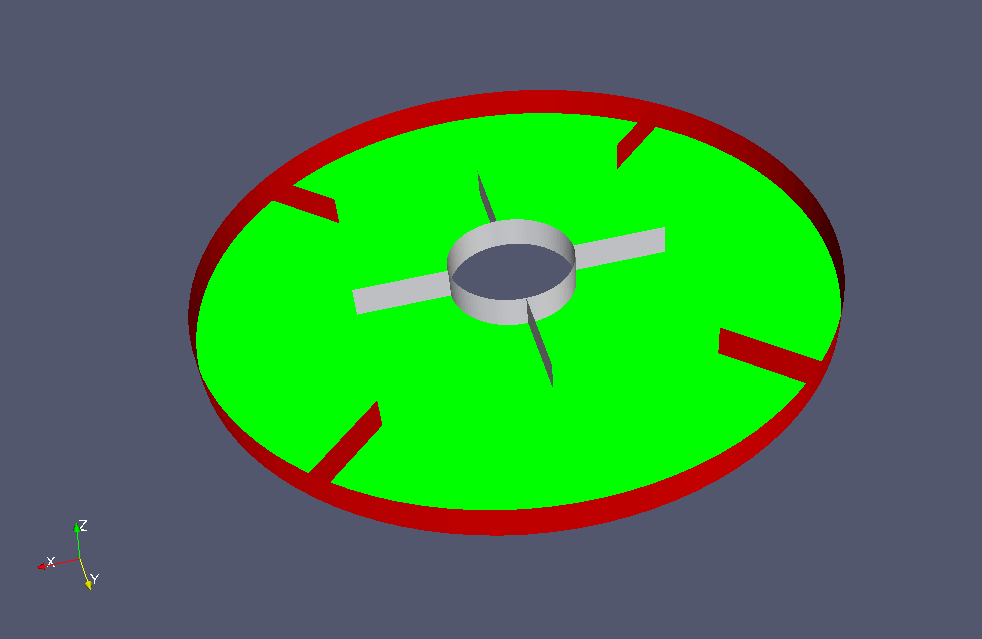Model geometry

The physical properties and interactions of each phase are defined in the files "constant/thermophysicalProperties.*" and "constant/phaseProperties", respectively.

The rotation axis and the rotation speed are defined in the file "constant/MRFProperties" as follows.

MRF1
{
cellZone    rotor;
active      yes;

nonRotatingPatches ();

origin    (0 0 0);
axis      (0 0 1);
omega     constant 10.472;
}

The rotation area is defined as the cell zone "rotor" as shown in the figure below (white part).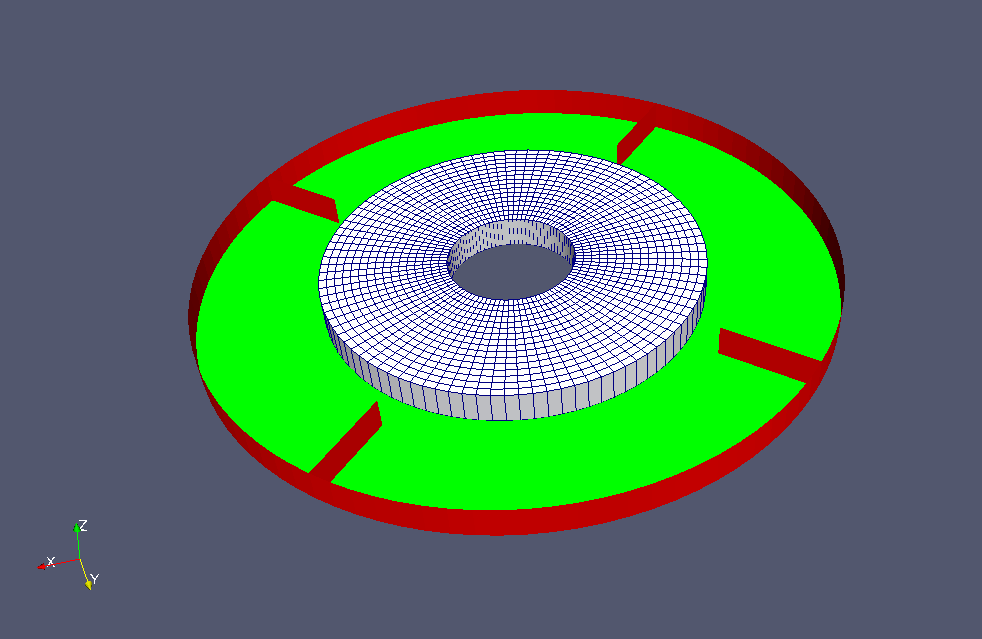Cell zone "rotor"

The meshes are as follows, and the number of mesh is 3072.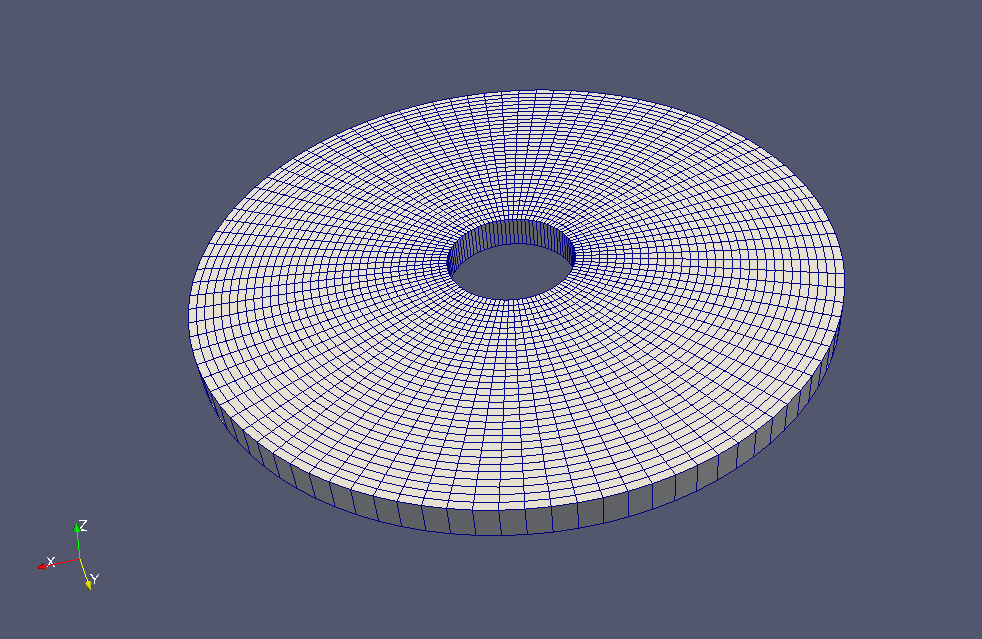Meshes

The calculation result is as follows.

Volume ratio of air (alpha.air)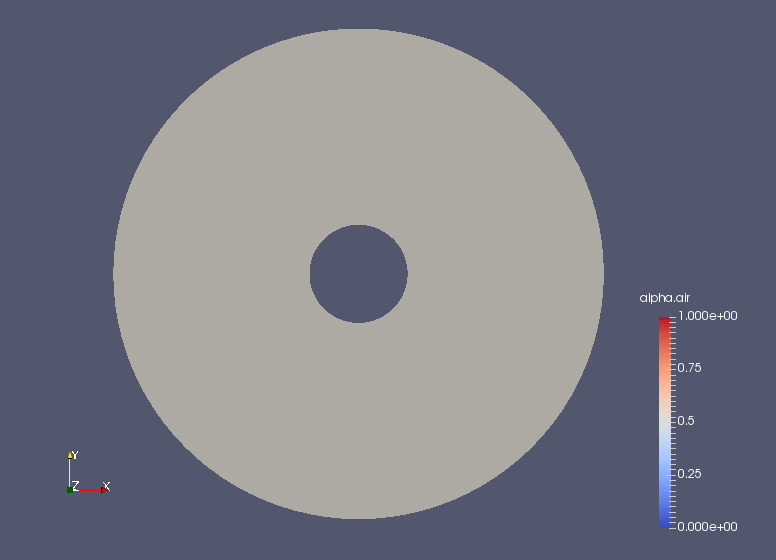Volume ratio of air at initial time (alpha.air)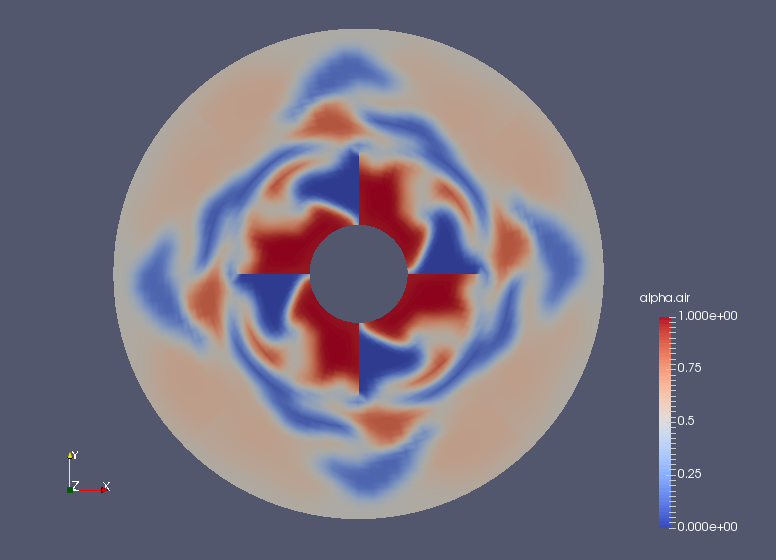Volume ratio of air at 0.3 sec (alpha.air)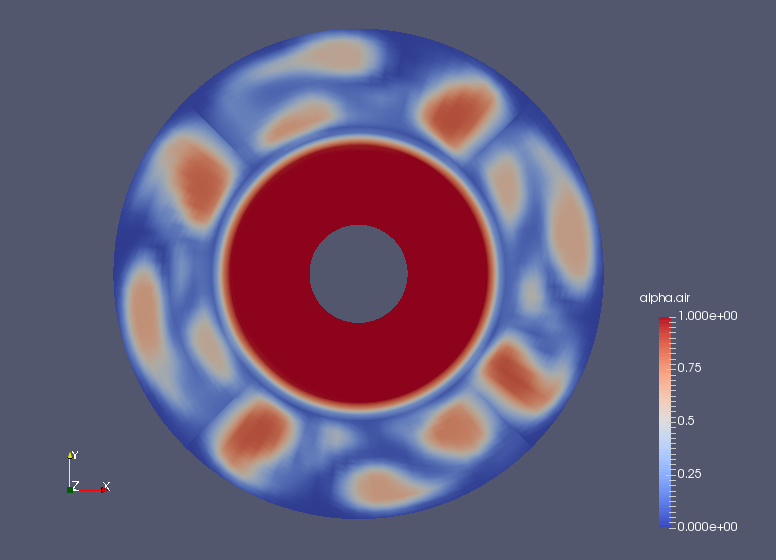Volume ratio of air at 10 sec (alpha.air)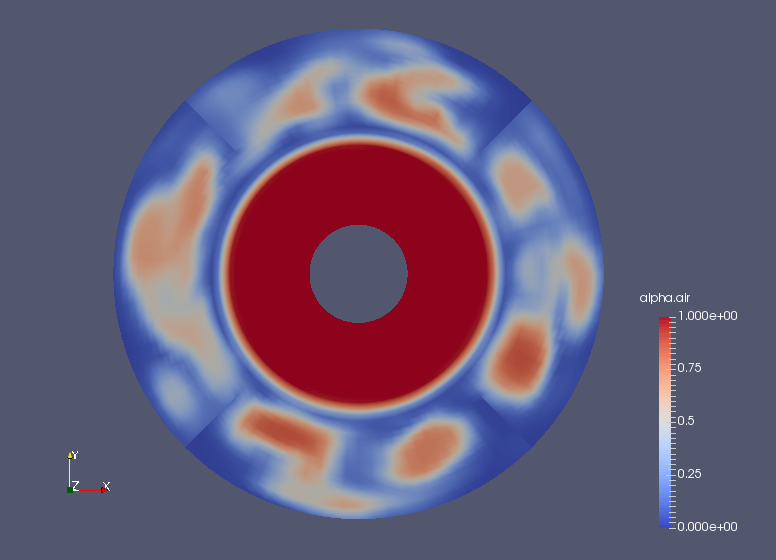Volume ratio of air at final time (alpha.air)

Commands

cp -r \$FOAM_TUTORIALS/multiphase/twoPhaseEulerFoam/laminar/mixerVessel2D mixerVessel2D
cd mixerVessel2D

m4 < system/blockMeshDict.m4 > system/blockMeshDict
blockMesh
topoSet
setsToZones -noFlipMap

twoPhaseEulerFoam

paraFoam

Calculation time

24 minutes 12.64 seconds *Single, Inter(R) Core(TM) i7-2600 CPU @ 3.40GHz 3.40GHz